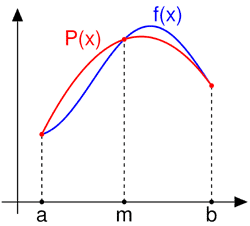I have forgotten

•http://facebook.com/
•https://www.google.com/accounts/o8/id
•https://me.yahoo.com
COST (GBP)3.000.000

# simpson

Computes the definite integral of a function using Simpson's first rule.
Controller: CodeCogsC++
HTML

## Overview

This module computes the area beneath either a user supplied function or a set of discrete points, using an approximation which assumes the function is shaped as a parabola between each two consecutive points.

{The background derivation of Simpon's first rule is given here:} Simpon's first rule represents the function between as a second order polynomial. The function is described by three ordinates, , and . In order to identify the truncation error, a fourth order polynomial is used,

Once integrated this becomes,

From (1), and

Therefore or

Substituting into (2),

If we ignore the last term, this would create a truncation error proportional to the fourth derivative of the function f(x), which depends on .

Consider a four times differentiable function , two distinct abscissas and a positive integer . Then the following approximation holds:

where , with an error bound of:

This is a quadrature formula known as Simpson's rule. From the formula of the error bound you may notice that e.g. if one doubles the number of points n, the approximation error is decreased sixteen times. This rule approximates the area under f between a and b by the area under a quadratic polynomial P as seen in the following image, where m is the midpoint between a and b.Below you will find examples that show how to use each version of the function and the error estimates in each case. Generally this module provides better estimates than using the trapezoidal rule as implemented in Quadrature/trapezoidal. To convince yourself, compare the approximations generated by this module with the estimates given by the trapezoidal rule module.

## References:

### Authors

Lucian Bentea (September 2006)

## Simpson

 doublesimpson( int n double (*f)(double)[function pointer] double a double b )
This version is to be used when you wish to pass the function to integrate as an argument, instead of passing an array with its values at distinct points.

### Example 2

In what follows an approximation is found for the definite integral and the absolute error from its actual value is estimated.
#include <codecogs/maths/calculus/quadrature/simpson.h>
#include <stdio.h>
#include <math.h>

// number of points
#define N 100

// function to integrate
double f(double x)
{
return sin(x);
}

// the primitive of f, to estimate errors
double pf(double x)
{
return -cos(x);
}

int main()
{
// compute the approximate area
double fi = Maths::Calculus::Quadrature::simpson(N, f, 1, 3),

// use the Leibniz-Newton formula to find a more precise estimate
realfi = pf(3) - pf(1); // uses the Leibniz-Newton formula

// display problem data
printf("      f(x) = sin(x)\n");
printf("    points = %d\n\n", N);

// display the result and error estimate
printf("   I(1, 3) = %.15lf\n", fi);
printf("real value = %.15lf\n", realfi);
printf("     error = %.15lf\n\n", fabs(fi - realfi));

return 0;
}

### Output

f(x) = sin(x)
points = 100

I(1, 3) = 1.530294803828911
real value = 1.530294802468585
error = 0.000000001360326

### Parameters

 n the number of sample points of the function f, from which to approximate (must be even) f the function to integrate a the inferior limit of integration b the superior limit of integration

### Returns

The definite integral of the given function from a to b.
##### Source Code

Source code is available when you buy a Commercial licence.

Not a member, then Register with CodeCogs. Already a Member, then Login.

## Simpson

 doublesimpson( int n const double* values double a double b )
This version is to be used when the function is not known analytically, but a table with its values at equally spaced abscissas is available.

### Example 3

The code below gives an approximation for the definite integral and estimates the absolute error from its actual value.
#include <codecogs/maths/calculus/quadrature/simpson.h>
#include <stdio.h>
#include <math.h>

// the primitive of g, to estimate errors
double pf(double x)
{
return 2*sqrt(x*x*x)/3;
}

int main()
{
// values of the function at equally spaced abscissas
double ordinates = {1, 1.0247, 1.04881,
1.07238, 1.09544, 1.11803, 1.14017},

// compute the approximate area
fi = Maths::Calculus::Quadrature::simpson(7, ordinates, 1, 1.3),

// use the Leibniz-Newton formula to find a more precise estimate
realfi = pf(1.3) - pf(1); // uses the Leibniz-Newton formula

// display problem data
printf("      f(x) = sqrt(x)\n");
printf("    points = 7\n\n");

// display the result and error estimate
printf(" I(1, 1.3) = %.15lf\n", fi);
printf("real value = %.15lf\n", realfi);
printf("     error = %.15lf\n\n", fabs(fi - realfi));

return 0;
}

### Output

f(x) = sqrt(x)
points = 7

I(1, 1.3) = 0.321485166666667
real value = 0.321485368419253
error = 0.000000201752586

### Parameters

 n the number of sample points of the function f, from which to approximate (must be odd) values an array with the value of the function at equally spaced abscissas a the inferior limit of integration b the superior limit of integration

### Returns

The definite integral of the given function from a to b.
##### Source Code

Source code is available when you buy a Commercial licence.

Not a member, then Register with CodeCogs. Already a Member, then Login.